## Flyback converter snubber design

##### Introduction

The objective of this experiment is to design a snubber circuit for flyback converter to clamp the voltage across the switch.

##### Theoretical background

Unlike the case of an ideal flyback converter waveform discussed in the previous experiment, Flyback converter, the voltage across the transistor of a practical flyback converter has significant ringing due to the parasitic elements.The energy stored in the transformer leakage inductance and the PCB trace inductance, Ll, unlike the energy stored in the magnetizing inductance Lm, does not transfer over to the secondary side when the transistor is turned off. This energy charges up the transistor’s parasitic capacitance, leading to a high voltage across it, causing significant voltage stress, which can damage the transistor, the transformer insulation, or both.

In a practical flyback converter, the magnitude of the voltage swing is clamped by the use of a snubber circuit.The switching cycle waveform of the snubber is shown below. The voltage across the snubber capacitor is assumed to be a constant Vcs, which is justified given a sufficiently large Cs. At time 0 < t < dTs, when the transistor is on, the primary current increases linearly. During this period, the voltage across the transistor is zero, and there is no current flowing into the snubber circuit because the diode Ds is reverse biased with a voltage of Vin + Vcs. across it. The snubber capacitor is slowly discharged by the snubber resistor, as shown by the negative current, −VCs / Rs.When the transistor is turned off, the parasitic capacitance across the transistor is charged up to the sum of the input voltage and the reflected secondary voltage, Vin + (N1 / N2) × Vo. During this interval, dTs < t < T1, the energy stored in the magnetizing inductance contributes to a rapid charging of the transistor’s parasitic capacitance, as well as the discharging of the secondary side diode’s junction capacitance. The input current iin remains relatively constant, given the negligible energy transfer from the magnetizing inductance to the parasitic capacitances.

Once the secondary side diode’s junction capacitance is fully discharged, and the diode begins conducting and simultaneously transistor parasitic capacitance is charged to Vin + (N1 / N2) × Vo, the energy stored in the magnetizing inductance starts charging the output capacitor.

During the time interval T1 < t < T2, the snubber diode is still reverse biased since (N1 / N2) × Vo < VCs. The voltage across the transistor continues to rise until it reaches Vin + VCs, plus the diode forward voltage drop, which is assumed to be negligible), forward biasing the snubber diode. The primary current during this interval falls rapidly as only the energy stored in the primary side leakage inductance is available to charge the transistor parasitic capacitance.

In the absence of the snubber circuit, the energy in the leakage inductance continues to charge the transistor parasitic capacitance, which leads to high voltage ringing across the transistor. In addition to the issue of high voltage, the ringing frequency is typically in the order of MHz and is a source of significant noise and EMI.

In the presence of the snubber circuit, the primary current flows through the forward-biased snubber diode, charging the snubber capacitor. VCs. VCs remains relatively constant by design, as explained in the following section. During this interval, T2 < t < T3, the primary current continues to fall rapidly. The voltage across the transistor remains clamped at Vin + VCs.

Once the leakage energy is fully transferred to the clamp capacitor at time t = T3, the primary current goes to zero, and the snubber diode gets reverse biased as the transistor voltage drops from Vin + VCs to Vin + (N1 / N2) × Vo.

RCD snubber design

At steady state, since the voltage across the snubber capacitor is a constant, the current through the capacitor averaged over a switching cycle is zero.

12 × Ipk × (T3 - T2) - VCsRs × Ts = 0(1)

During the time interval T2 < t < T3, when the snubber diode is forward biased, the voltage across the parasitic inductor Ll is the sum of the voltage across the snubber capacitor and the voltage across the transformer primary winding.

VLl = Ll × ∆Ipri∆t = VCs + n × Vo(2)

where, n = N1 / N2

The current through the leakage inductor goes from approximately Ipk to zero. The duration of the interval when the snubber diode is conducting can be obtained from Eqn. 2

Ll × ∆Ipri∆t = Ll × Ipk - 0T3 - T2 = VCs + n × Vo

⇒ Tsn= T3 - T2 = Ll × IpkVCs + n × Vo(3)

The expression for the snubber resistor is be obtained using Eqns. 1 and 3

12 × Ipk2 × Ll × 1VCs + n × Vo - VCsRs × Ts = 0

⇒ Rs = VCs × (VCs + n × Vo)0.5 × Ipk2 × Ll × fs(4)

where, fs = 1 / Ts.

Given the converter operating condition and the desired maximum transistor voltage, the snubber resistor can be determined using the above expression. The lower the tolerable voltage across the transistor, the smaller the snubber resistor and thus higher losses in the snubber circuit. The power lost in the snubber circuit is:

Ps = VCs2Rs = 12 × Ipk2 × Ll × fs × VCsVCs + n × Vo = α × PLl(5)

where,

Ps = VCs2Rs = 12 × Ipk2 × Ll × fs(6)

α = VCsVCs + n × Vo(7)

PLl is the leakage inductor power, the energy stored in the leakage inductor per switching cycle time period. α is the factor by which the losses in the circuit are scaled due to the presence of the snubber compared to the circuit without a snubber. Since VCs < (N1 / N2) × Vo, α is always greater than 1. The higher the snubber voltage, the lower the losses and vice versa.

The value of the snubber capacitor isn’t as crucial as that of the resistor. The only requirement is that the capacitance is large enough to maintain the snubber voltage a constant. An approximate expression can be obtained from the snubber capacitor current waveform in the above image. Assuming that the current in the snubber resistor is negligible compared to the peak input current Ipk, during the interval when the snubber diode is reverse biased

iCs = Cs × ∆VCs∆t

VCsRs = Cs × ∆VCsTs - Tsn

⇒ Cs = 1Rs × VCs∆VCs × (Ts - Tsn) ≈ TsRs × VCs∆VCs(8)

In the above expression ∆VCs / VCs is the ratio of the snubber capacitor peak-peak ripple voltage and the snubber capacitor average voltage over a switching cycle. This value is typically chosen between 0.01 and 0.1.

The diode must be a fast-acting diode such as the Schottky diode since the conduction period Tsn typically lasts for a short interval.

In the following section, Flyback converter experiment is repeated with the only difference being that the jumper on the magnetics board is removed to disconnect the snubber. This would lead to significant ringing in the voltage across the transistor. The results are compared with the those obtained in the previous experiment with the snubber connected.

##### Preparing the Workbench model
1. Copy the folder where pre-built example project for this experiment is present, usually in C:\Program Files (x86)\Sciamble\WorkBench v1\Examples\CUSPLab\BasicPowerElectronics\Experiment12 and paste it in a location where the user has permission to edit and save files, like the Desktop folder.

2. Launch Workbench application.

3. Pin (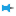) the Explorer dock on the right and the Toolbox dock on the left.

4. Click the second icon within the Explorer dock,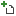to open the project.

5. Navigate to the folder where the pre-built example project was pasted in Step 1. Double click the FlybackConverter.project node within that folder to open the project.

6. Click the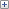icon to explore the files within the project. Double click the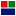Flyback node in the Explorer dock to display the model file.

7. Ensure that the switching frequency is set to 100 kHz. To do this, double click on the project node,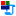BuckBoostConverter, in the Explorer. Go to the next page by clicking the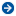in the property dock or choosing Device configuration from the drop-down menu. Check that the Frequency within PWM Configuration is set to 100000.

8. Ensure that the PWM channel is set to the Si power pole. To do this, open the property of the tool labelled Si PWM in the model by double clicking on it. Check that the Channel is set to 1.

##### Preparing the setup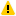Warning

Before proceeding, ensure that the isolated power supply is powered down and that the USB cable is disconnected.

1. Magnetics card connection: Unscrew any existing magnetics card and replace it with the Flyback magnetics card. Make sure that all the 6-pins of the magnetics card are making solid contact with the power-pole board.

Prior to connecting the card, ensure that the jumper on the bottom of the magnetics card is disconnected. This is to disconnect the snubber circuit across the transformer primary to observe the real response of the converter in the absence of the snubber circuit.Warning

Never leave the magnetics card unscrewed. If contact is lost while the converter is running, it has the potential to cause very high voltage due to interruption of inductor current, potentially damaging the converter, or worse, could lead to a safety hazard.

2. Rheostat setting: Set the slider such that the resistance across the two closest rheostat terminals is 8 Ω.

3. Power connections:

1. Connect the Vin terminals to the isolated power supply:

1. DC +ve: Vin+ (Red)

2. Ground: Earth (Green)

3. `DC −ve: ● Vin− (Black)`

2. Connect the Vo terminals to the rheostat load:

1. Rheostat rail: Vo+ (Red)

2. `Rheostat's other terminal that is closest to the rail: ● Vo− (Black)`

4. DSO connections:

1. Connect DSO channel 1 probe to Io. Set the following options, if they are supported by the DSO:

1. Set the probe to 1x.

2. Set the measurement type as Current.

3. Set the scaling factor to 5x.

4. Set the offset at 1.5 V or 7.5 A.

5. Set termination to 1 MHz.

6. Set the channel as inverted.

2. Connect DSO channel 2 probe to SWA. Set the following options, if they are supported by the DSO:

1. Set the probe to 1x.

2. Set the measurement type as Voltage.

3. Set the scaling factor to 1x.

4. Set the offset at 0 V.

5. Set termination to 1 MHz.

6. Set the channel as default/non-inverted.

3. Connect DSO channel 3 probe to Iin. Use the same setting as that of Channel 1.

4. Connect DSO channel 4 probe to Vout. Use the same setting as that of Channel 2.

5. Jumper settings:

1. Disconnect the Vdc jumper.

2. Insert the GaN/Si jumper to the Si side.

3. Insert the GaN switch's external diode jumper, labelled "Diode".

4. The jumper on the bottom of the magnetics card was removed in Step 1, while inserting the card.

6. Connect the USB cable to the power-pole board and the computer.

7. DC power supply settings:

1. Make sure that the DC power supply is fully turned down to 0 V prior to turning on the supply.

2. Turn on the power supply and gradually ramp up the voltage from 0 V to 15 V.

3. If the option is available, set the power supply current limit at 4.5 A.

The final wiring should look similar to this: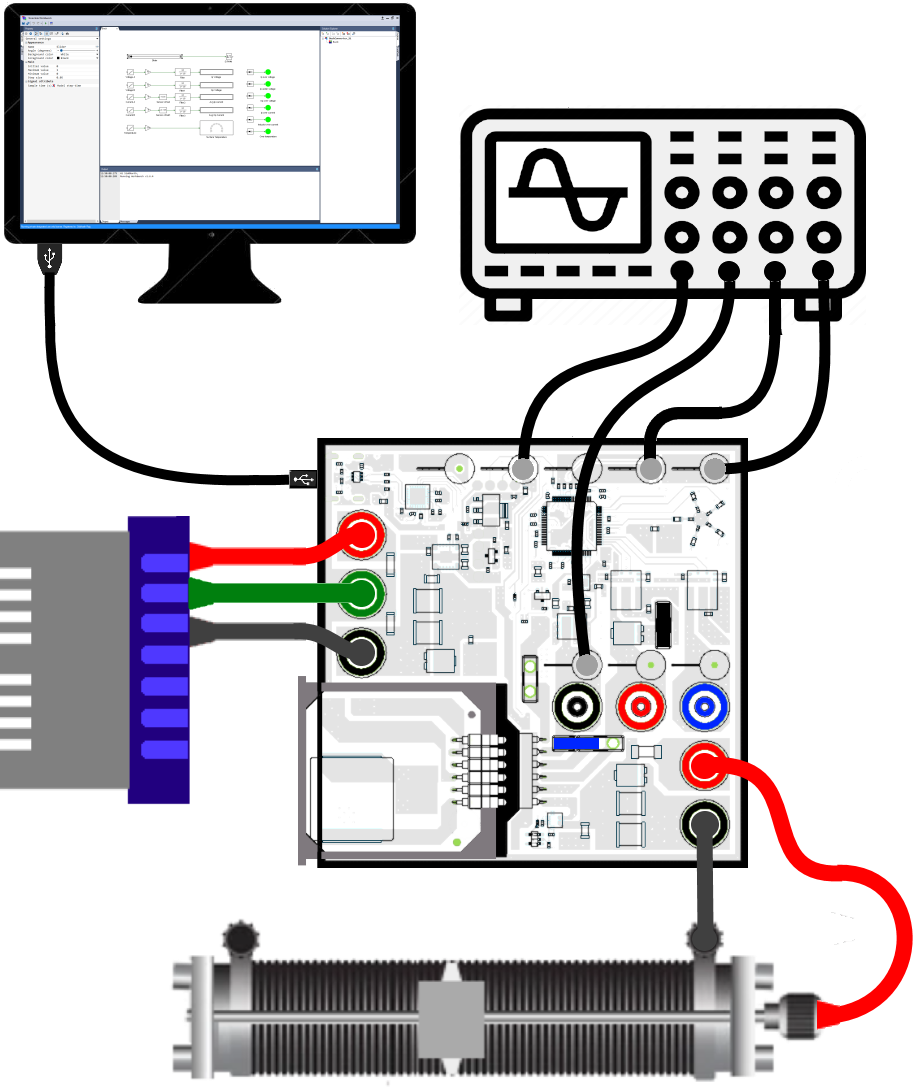Warning

The input and output of a typical flyback converter is galvanically isolated. That is not the case in this lab kit.To enable both external measurement as well as measurment by the controller of the input, the output, and the switch voltages without the need for measurement isolation, the output negative is internally connected to the input negative. Thus the output is not electrically isolated. This does not alter the response of the converter in any manner.

##### Real-time open loop control of flyback converter - with snubber
Running the setup:
1. Click on the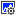icon in the top dock of Workbench to transition from the simulation mode to the real-time mode.

2. Click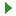to run the control algorithm in real-time.

3. If an undervoltage fault occurs, slightly increase the input voltage above 15 V but less than 16 V. Stop the model by clicking on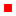and rerun it.

4. Compensate the offset in the current sensors. To do this, make note of the value, up to 3 decimal places, displayed on the Avg Ip Current and the Avg Op Current tool.

5. Click onto stop the model.

6. Open Sensor offset tool's property by double clicking on it and set the Offset value to the negative of the measured Avg Ip Current in the previous step.

Open Sensor offset1 tool's property and set the Offset value to the negative of the measured Avg Op Current in the previous step.

7. Clickto rerun model.

8. Once programmed, ensure that the Avg Ip Current and the Avg Op Current both initally display a value of 0 for up to 2 decimal places. If not, repeat the previous 3 steps.

9. Gradually increment the duty cycle from 0 to 0.5 in steps of 0.05. If a fault occurs, stop the model by clicking onbutton.

10. Make the following measurements:

1. Observe the DSO waveforms and make a copy of the voltage across the switch (channel 2), the output voltage (channel 4), the input/primary current (channel 1), and the diode/secondary current (channel 3) for duty cycle of 0.5 (or the highest possible value prior to when the fault occured). Adjust the time base to show anywhere between 4-10 switching cycles.Warning

Do not exceed duty cycle of 0.5. Even though the input and output voltages, which are monitored by the controller, will be within safe limits, the switch voltage, which is not monitor by the controller, could exceed the device rating for duty greater than 0.5. This is because the voltage across the switch is the sum of input voltage and the reflected output voltage. In addition, the absence of the snubber circuit leads to significant ringing causing even higher voltage stress across the transistor.

11. Click onto stop the model.

##### Lab report and reading assignment
1. Attach the DSO waveforms showing the voltage across the transistor, the output voltage, the inductor current, and the input current for switching frequency of 100 kHz and duty cycles of 0.5.

2. Compare the results obtained with the snubber (previous experiment) and without the snubber (current experiment).

3. Design a RCD snubber for the flyback converter using the operating conditions and parameters determined in the previous experiment. Assume that the snubber diode is ideal and the peak input current is Ipk = 2 A. The voltage across the transistor should not exceed 35 V under the given operating conditions.

##### Reference
1. "Power Electronics, A First Course," Ned Mohan and Siddharth Raju, Wiley Publication.

< Flyback converter : previous topic
next topic : Forward converter >